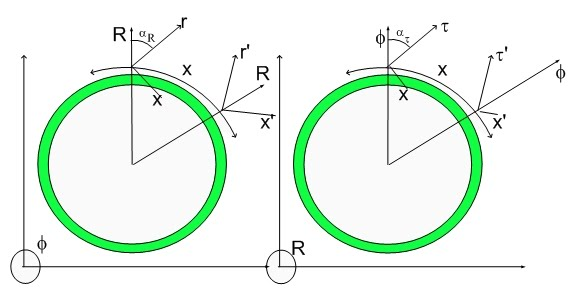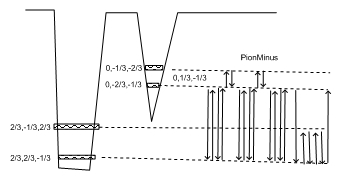# Hypergeometrical Universe

## Pion Decay DetailsPion Minus Decay Details

I should repeat the same detailed description I created for the Delta decay.

A complex coherence sequence with multiple fundamental dilator chords is a polymer, in the sense that its 3D representation seemingly contains more than one particle. In reality, one should be able to see three particles (does that rings a Bell someplace) in the case of a Pion Minus, two in the case of a Neutron...

The decaying reaction for the trimeric Pion Minus can be redrawn as:Please click the picture to see a larger version of it. The left side fo the decaying equation is the Pion Minus coherence followed up to six Fundamental Dilator Cycles (FDC) This is because the Pion Minus is a trimer thus it requires three FDC to cover a full cycle while the Muon Neutrino Transient State is a dimer. The minimum common multiple is six Fundamental Dilator Cycles.

Of course, each Balls Diagram has a corresponding state diagram. The Pion Minus corresponds to the following coherence:Reading the coherence from left to right, one can identify:
1) Electron Fundamental Dilator
2) Half-Muon Neutrino (Electron-Positron Transmutation Chord - the blue line).
3) Positron Fundamental Dilator
4) Half-Muon Neutrino (Electron-Positron Transmutation Chord - the blue line).
5) Electron Fundamental Dilator
6) Electron - Proton Transmutation Chord followed by an Proton-Electron Transmutation Chord. This is equivalent to an Electron Neutrino.

Just for sake of being throrough let's revise the Neutron Balls and Coherence Diagrams.and its Coherence Diagram:Reading the coherence from left to right, one can identify:
1) Electron Fundamental Dilator
2) Half ElectronNeutrino (Electron-Proton Transmutation Chord - the red line).
3) Proton Fundamental Dilator
4) Half ElectronNeutrino (Electron-Proton Transmutation Chord - the red line).
6) Electron - Proton Transmutation Chord followed by an Proton-Electron Transmutation Chord. This is equivalent to an Electron Neutrino.

The only three construct that one requires to understand are the Fundamental Dilator, the Electron-Proton Transmutation Chord and the Electron-Positron Transmutation Chord. All Hyperons, and isotopes are just combinations of those three elements.

Of course, isotopes are even simpler. They only have protons and neutrons or fundamental dilators and Electron-Proton and Proton-Electron Transmutation Chords. Since isotopes are complex coherences, their 3D representation is a very, very complex tridimensional rotating object composed of many fundamental dilators.

Cheers,

MP

Currently unrated

or